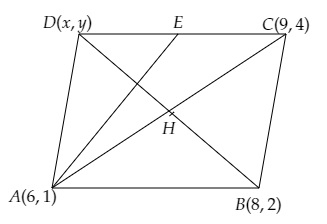# $A (6, 1), B (8, 2)$ and $C (9, 4)$ are three vertices of a parallelogram $ABCD$. If $E$ is the mid-point of $DC$, find the area of $\triangle ADE$.

Given:

$A (6, 1), B (8, 2)$ and $C (9, 4)$ are three vertices of a parallelogram $ABCD$.

$E$ is the mid-point of $DC$.

To do:

We have to find the area of $\triangle ADE$.

Solution:

Let the fourth vertex of the parallelogram be (x, y).We know that, the diagonal of a parallelogram bisect each other.

The mid-point of a line segment joining the points $(x_{1}, y_{1})$ and $(x_{2}, y_{2})=(\frac{x_{1}+x_{2}}{2}, \frac{y_{1}+y_{2}}{2})$

Mid-point of $\mathrm{BD}=$ Mid point of $\mathrm{AC}$

$\Rightarrow (\frac{8+x}{2}, \frac{2+y}{2})$

$=(\frac{6+9}{2}, \frac{1+4}{2})$

$\Rightarrow (\frac{8+x}{2}, \frac{2+y}{2})=(\frac{15}{2}, \frac{5}{2})$

On comparing, we get,

$\frac{8+x}{2}=\frac{15}{2}$

$\Rightarrow 8+x=15$

$\Rightarrow x=15-8=7$

$\frac{2+y}{2}=\frac{5}{2}$

$\Rightarrow 2+y=5$

$\Rightarrow y=5-2=3$

The fourth vertex of the parallelogram is $\mathrm{D}(7,3)$.

The mid-point of side $\mathrm{DC}=(\frac{7+9}{2}, \frac{3+4}{2})$

$E=(8, \frac{7}{2})$

The area of $\Delta \mathrm{ABC}$ with vertices $(x_{1}, y_{1}),(x_{2}, y_{2})$ and $(x_{3}, y_{3})=\frac{1}{2}[x_{1}(y_2-y_{3})+x_{2}(y_{3}-y_{1})+x_{3}(y_{1}-y_{2})]$.

This implies,

Area of $\Delta \mathrm{ADE}$ with vertices $\mathrm{A}(6,1), \mathrm{D}(7,3)$ and $\mathrm{E} (8, \frac{7}{2})$

$=\frac{1}{2}[6(3-{7}{2})+7(\frac{7}{2}-1)+8(1-3)]$

$=\frac{1}{2}[6 \times(\frac{-1}{2})+7(\frac{5}{2})+8(-2)]$

$=\frac{1}{2}(-3+\frac{35}{2}-16)$

$=\frac{1}{2}(\frac{35}{2}-19)$

$=\frac{1}{2}(\frac{-3}{2})$

$=\frac{-3}{4}$

Area cannot be negative.

Hence, the required area of $\Delta \mathrm{ADE}$ is $\frac{3}{4}$ sq. units.

Updated on: 10-Oct-2022

37 Views